• 在之前的文章中，我们已经解释了向量可以写成[1 x 3]的矩阵（一）。但是现在也可以写成[3 x 1]（三）的形式。从技术上来说，这两种表示点和向量的方式都是合理的，选用一种模式只是涉及到习惯的问题。 ...
在之前的文章中，我们已经解释了向量可以写成[1 x 3]的矩阵（一行三列）。但是现在也可以写成[3 x 1]（三行一列）的形式。从技术上来说，这两种表示点和向量的方式都是合理的，选用一种模式只是涉及到习惯的问题。
向量写成[1 x 3]的矩阵 V = [x  y  z]
向量写成[3 x 1]的矩阵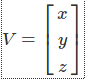在第一个例子中，我们写了一个[1 x 3]的矩阵，我们称之为行顺序（row-major order）：向量被写作一行三个数据。在第二个例子中，我们称点或者是向量为列顺序(column-major order)：点或者是向量的三个坐标被写成一列。
记住我们表达点或者是向量使用矩阵去乘以一个[3 x 3]的变换矩阵（为了简单起见我们使用[3 x 3]的矩阵而不是[4 x 4]的矩阵）。我们也学习到只有左边矩阵的列数等于右边矩阵的行数的时候这两个矩阵才可以相乘。换句话说也就是[m x p][ p x n]的两个矩阵可以相乘，而[p x m][p x n]的两个矩阵不可以相乘。记住我们可以将一个向量写成[1 x 3]，那么它可以和一个[3 x 3]的矩阵相乘，但是如果我们把这个向量写成[3 x 1]那么我们不能和一个[3 x 3]的矩阵相乘。这个道理会在下面的例子中显示。绿色的维数是一样的所以这个乘积是合法的（所得到的结果就是一个变换的点的坐标，它的形式是一个[1 x 3]的矩阵）：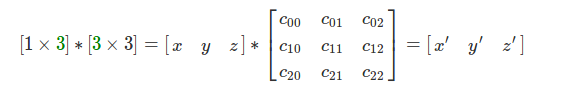里面的维数为(1和3)的两个矩阵在乘法中国因此这个成绩是不可能实现的：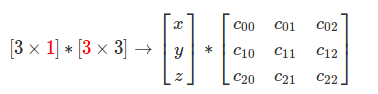那么我们应该怎么办？这个问题的答案不在是向量或者点去乘以矩阵，而是矩阵M去乘以向量V。换句话说，我们将点移动，或者向量移动到乘法的右边：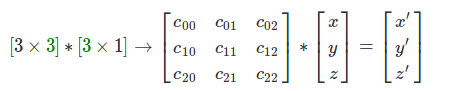记住这个操作所得到的移动的点写成[3 x 1]的矩阵方式。因此我们可以用我们想要的方式去获得一个变换的点。问题解决了，总结一下，当习惯的时候我们表达点或者向量是用的行顺序[1 x 3]，我们需要将点防止在乘法的左边，[3 x 3]的矩阵应该放置在乘法的右边。在数学上，这叫做左或者提前乘法（pre-multiplication）.如果你决定将向量写成列顺序，那么点应该放置在乘法的右边，这个叫做右或者是后置乘法（post-multiplication）.
我们应该对这些东西的使用格外的小心。比如，在Maya中，矩阵是后置乘法的，比如将一个点p从物体坐标系中移动到世界坐标系中，你需要的是后置的乘法P = P x WM.这是很迷惑人的，因为他实际上是提前乘法，但是他们说这个是以矩阵的位置来确定这个乘法的。在术语上这个实际上是不准确的。在Maya中，点和向量应该表达称行顺序，因此它们是属于提前乘法的（意味着点和向量出现在矩阵的之前）。
下面的这个表格总结了这两种惯例的不同之处（P表示点，V表示向量，M代表矩阵）。
行顺序  P / V = [x y z] 左或者前置乘法  P / V * M
列顺序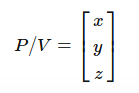右或者是后置乘法  M x P / V
现在我们学习了两种常规表达方式，你可能会问，为什么不把它们写在纸上？我们知道如何计算两个向量的乘积：A向量的当前行乘以相对应的B向量的当前列然后将这些结果相加。让我们运用这个公司去比较它们的结果：
行顺序（row-major order）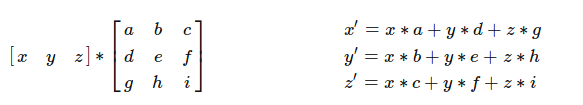列顺序（column-major order）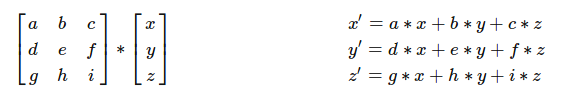点或者是向量和矩阵相乘不论是用行顺序还是列顺序我们都应该得到相同的结果。如果你在一个3D应用中，我们将一个点绕Z轴旋转一定的角度，你期望通过旋转之后的点在确定的位置上，不论开发者是用什么样的的方式表达点或者向量。但是，当你看到上面的表格之后，乘以一个行顺序或者是列顺序的矩阵我们不会得到相同的结果。回到我们的原点中，我们需要转置一个[3 x 3]的矩阵使它用在列向量中，从而确保x', y', z'是一样的结（如果你需要知道矩阵的转置是什么，请看矩阵操作章节），这里我们得到的：
行顺序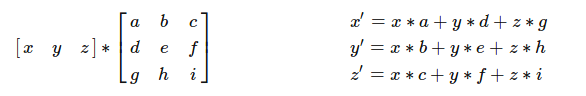列顺序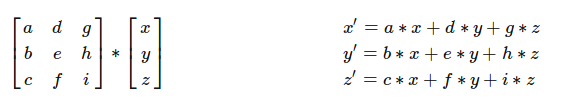总结来说，从行顺序到列顺序中不仅仅是变换了点和向量相对于乘法的位置而且将[3 x 3]的矩阵转置，这样做确保两个得到的结果是一样的。
从这些观察中可以得到，你可以将一系列的变换应用于一个点，当你使用行顺序的时候它们可以被写成一个阅读的顺序。比如你想将点P通过点T平移，然后绕Z轴旋转，然后绕y轴旋转。你可以写成下面的样式：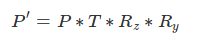如果你使用一个列顺序的话，你需要调用这些变换使用相反的顺序，如下面的样子：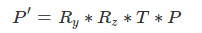因此你可能回想，这里肯定有一个原因更会喜欢一种。实际上，这两种习惯都是正确的，会给我们相同的答案，但是因为一些技术上的原因，数学和物理偏向于使用列向量。
使用列向量进行变换个何如我们写的数学公式。
行矩阵可以使得我们的矩阵更简单，因此对于这个教学网站来说我们使用它（和Maya， DirectX是一样的它们也在标准手册中定义了相关习惯）。但是对于另外一些3D的api,比如opengl， 使用的是列顺序习惯。
（1）隐式编程：它影响性能吗？
这里有一个潜在的很重要的需要考虑的当你使用行顺序或者是列顺序的时候，但是这个对于真正的习惯并没有什么用处，哪个更实用。它有跟多的使用电脑的工作方式。记住我们将会处理[4 x 4]的矩阵，典型的C++实现的矩阵是下面这个样子：

class Matrix44 {
float m
}你可以看到16个矩阵的参数存储在二维数组里面（如果你想选用双精度的，你可以使用模板类）。那么16个参数会以下面的方式进行排列：c00, c01, c02, c03, c10, c11, c12, c13, c20, c21, c22, c23, c30, c31, c32, c33.换句话说，他们在内存里是连续的。让我们来看看这些向量和矩阵的乘法是怎么样在行顺序中获取的：

// row-major order
x' = x * c00 + y * c10 + z * c20
y' = x * c01 + y * c11 + z * c21
z' = x * c02 + y * c12 + z * c22 你可以看到这些x'的元素不是线性获取的。换句话说，为了计算x'的值，我们需要第1， 5， 9个矩阵的数值。为了计算y'我们需要获取第2， 6， 10的数值。计算z'我们需要3, 7, 11的数值。在计算的世界里，不是线性第获取数组的元素不是一件好的事情。它实际上利用了cpu的缓存技术。我们不会去深入地研究，但是我们想说的是离cpu最近的缓存获取最快，但是它只能存储有限的数据。当cpu需要获取数据的时候，它首先检出数据是否存在缓存中。如果它存在，cpu就会马上获取他们，如果它不存在，首先cpu需要穿件一个缓存的入口，然后把内存中的数据复制到这里来。这个过程很显然地要比从缓存中直接读取数据花费更多的时间。cpu不仅会复制需要的数据，而且会复制一连串的数据，比如24个字节的数据。因为硬件工程师理解如果你的代码需要获取数组的元素，你更倾向于连续地获取。确实，在程序中，我们经常循环遍历玩数组的元素，这个假设因此是正确的。应用到我们的矩阵问题上，不连续地获取数组中元素当然是个问题。如果cpu允许三个浮点数的缓存，那么我们当前的实现就可能导致缓存没法命中。因为我们用于计算x', y', z'的参数是有5个分离。另一方面说，如果你使用的是列顺序，计算x'的值值需要获取1, 2,3个数值。

// column-major order
x' = c00 * x + c01 * y + c02 * z
y' = c10 * x + c11 * y + c12 * z
z' = c20 * x + c21 * y + c22 * z 矩阵的参数可以按顺序获取说明我们可以使用cpu的缓存技术。因此从编码的角度来说的话，进行点或者向量矩阵相乘的时候使用列顺序会比使用行向量更好。实际的，我们并没有展示这个例子（当你使用你优先级标志-O -O2 -O3编译你的程序的时候，编译器可以最优化你的循环），我们成功使用了行顺序的版本没有丢失任何的性能，相比较列向量来说。

template<typename T>
class Vec3 {
public:
Vec3(T xx, T yy, T zz) : x(xx), y(yy), z(zz) { }
T x, y, z, w;
};

#include <iostream>

#define ROWMAJOR 1

template<typename T>
class Matrix44 {
public:
T m;
Vec3<T> multVecMatrix(const Vec3<T> &v) {
#ifdef ROWMAJOR
return Vec3<T>(v.x * m + v.y * m + v.z * m,
v.x * m + v.y * m + v.z * m,
v.x * m + v.y * m + v.z * m);
#else
return Vec3<T>(v.x * m + v.y * m + v.z * m,
v.x * m + v.y * m + v.z * m,
v.x * m + v.y * m + v.z * m);
#endif
}
};

#include <cmath>
#include <cstdlib>
#include <cstdio>
#include <ctime>

#define MAX_ITER 10e8

int main(int argc, char **argv) {
clock_t start = clock();
Vec3<float> v(1, 2, 3);
Matrix44<float> M;
float *tmp = &M.m;
for (int i = 0; i < 16; i++)
*(tmp + i) = drand48();
for (int i = 0; i < MAX_ITER; ++i) {
Vec3<float> vt = M.multVecMatrix(v);
}
fprintf(stderr, "Clock time %f\n", (clock() - start) / float(CLOCKS_PER_SEC));
return 0;
}如果运行的话就会走row分支，因为ROWMAJOR之前已经有定义。

两者运行时间基本上一致

wang@wang:~/workspace/graphic$./multiMatrix Clock time 14.660049 wang@wang:~/workspace/graphic$ g++ -std=c++11 multiMatrix.cpp -o multiMatrix
wang@wang:~/workspace/graphic$./multiMatrix Clock time 14.597244列顺序稍微快那么一点点，不到0.1秒的差距。 （2）计算中的行顺序和列顺序 为了完整性，行顺序和列顺序可以用于计算中，用来描述多维数组的元素在内存中的分布。在行顺序中，多维数组的元素排列从左到右，从上到下。可以使用C/c++表示他们。比如，矩阵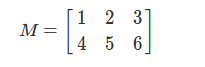可以写成代码的方式： float m={{1, 2, 3}, {4, 5, 6}}; 然后这些元素会在内存中线性排列： 1 2 3 4 5 6 在列向量中，它用在Fortran和matlab中，元素的在内存中的排列方式是从上到下，从左到右的。使用上面的矩阵，那么矩阵按照下面的方式存储： 1 4 2 5 3 6 知道矩阵中的元素在内存中是如何存放的是很重要的，因为有时候你会使用指针的偏移去获取他们。对于循环的优化（之前的小节中我们已经讲到它会影响到cpu的缓存性能）。但是，因为我们只考虑c/c++作为我们的编程语言，列顺序我们没有多大的兴趣。我们仅仅只是在计算中提到它们是什么，因此你可能会根据具体的语义去描述它们。你应该不能将它们混合。在数学上，它们描述你是用行向量，还是列向量。在计算上，它们描述了数据的储存或者获取方式。 Opengl是一个很有趣的例子，当GL初始化的时候，开发者选用行向量。开发者开发新功能的时候又要回到列向量中。但是，为了兼容性，它们不想改变点和矩阵的成绩，而是去改变矩阵在内存中存储的顺序。换句话说，opengl存储参数使用的列顺序， 参数m03, m13， m23,使用列向量会有13， 14， 14的下标识，而在行向量中有m30, m31, m32的标识。 （3）总结 下面对行向量和列向量这两种用法进行总结：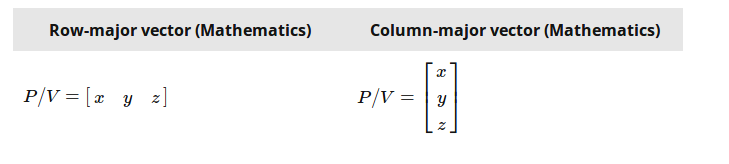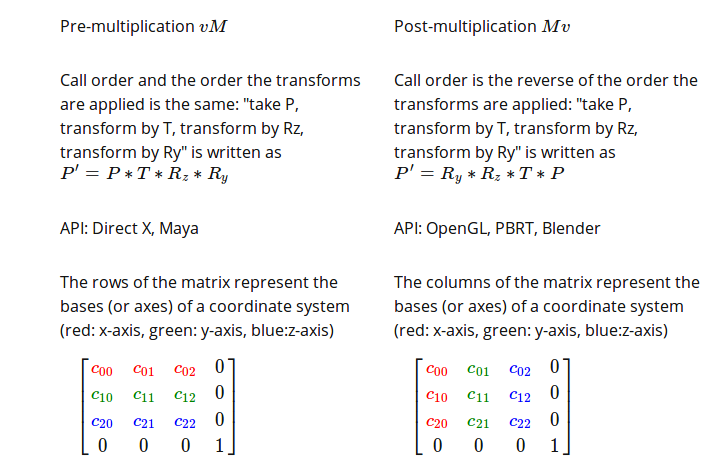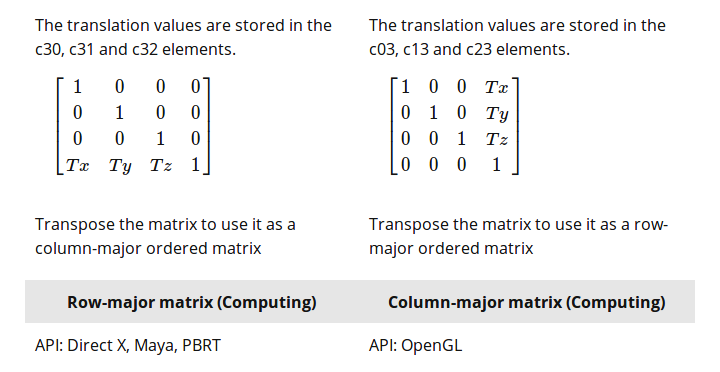上面的英语不难，应该能看懂。 一个肚子在Stackoverflow上提了一个上面表格的问题，认为它很容易使人迷惑。这个话题是迷惑的，尽管我们已经尽了最大的努力，许多人对于它们还是感到迷惑。我们希望我们在Stackoverflow上的回答可以让读者从另一个视角来看待这个问题。 你已经有理论，你现在要做的是用C++实现它，这里有两个不同的问题。 数学上的：你可以些两种方式，可以是列顺序也可以是行顺序，如果使用行顺序，你应该将向量和矩阵的乘法写成vM，这里的表示(1 x 4)的向量，M表示（4 x 4）的矩阵。因为只有写成[1 x 4]*[4 x 4]才能进行矩阵的计算。相似地如果你想使用列顺序，你的向量应该写成竖直的，[4 x 1]，因此矩阵的乘法应该写成这种样式：[4 x 4] *[4 x 1].矩阵防止在向量的前面Mv.前面一种方式叫做后置乘法，后面一种Mv叫做前置乘法（矩阵在前面）。 现在，你需要编一个一个向量或者是点，你需要注意矩阵乘法的顺序，当你把它们写在纸上的时候。如果你使用矩阵T进行平移，然后使用矩阵R进行旋转，然后使用S进行缩放变换，如果使用列矩阵的话，应该写成下面这个样式: v' = S * R * T * v。在行矩阵中你应该写成v' = v * T * R * S. 这是理论上的，我们把它叫做行/列矩阵常用习惯。 计算机上的：这关系到你如果用C++实现它们。好的消息是C++不会给你增加任何的显示。你可以将你的矩阵的参数用你想的方式放置它们，你可以自己些代码表示矩阵的乘法。相似的，你获取向量矩阵乘法的参数的操作也是取决于你。你应该做出一个清晰的区别你的矩阵参数如何放在内存中，以及你将使用那种数学习惯看待你的向量。这两个独立的问题，我们把这个叫做行/列分布。 比如，你可以定义个矩阵类有16个连续的浮点数据。那是好的，这里的参数，m14, m24, m34表示的矩阵平移的(Tx, Ty, Tz).因此这是一种列矩阵，尽然你被告知Opengl使用的矩阵是列矩阵。这里有一个很容易迷惑的就是将参数放置在内存中，不同于你实际想要表达的列矩阵。你写成行的形式，但实际上它们是列的，因此你不知道你时候在做正确的事情。 这里很重要的是，矩阵表示一个坐标系统的三根轴。哪里以及如何存储这些数据完全取决于你。想象一些三个向量表示坐标系统的三根轴，其中它们命名AX(x, y, z), AY(x, y, z), AZ(x, y,z)已经它们的平移向量表达称（Tx, Ty, Tz),那么从数学上你可以使用列向量表示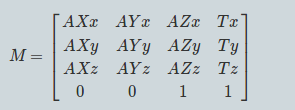这些轴被写成竖直的形式。现在你如果使用行向量，那么可以写成如下的形式：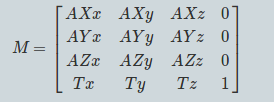它的坐标系统的轴是横向的。因此接下来的问题是你的电脑如何存储这些数据的问题。你可以用下面的形式： float m = { AXx, AXy, AXz, 0, AYx, AYy, AYz, 0, AZx, AZy, AZz, 0, Tx, Ty, Tz, 1};你也可以写成下面这样： float m = { AXx, AXy, AXz, Tx, AYx, AYy, AYz, Ty, AZx, AZy, AZz, Tz, 0, 0, 0, 1}; 或者这样： float m = { AXx, AYx, AZx, Tx, AXy, AYy, AZy, Ty, AXz, AYz, AZz, Tz, 0, 0, 0, 1}; 再次地高数你，选择什么的数学习惯你随便选择。你仅仅只是将16个参数通过不同的方式存储在内存中，只要你准确地知道它们是什么，因此你可以在后面获取它们。记在心里的是一个向量和一句矩阵相乘应该给你一个相同的行或者是列的数学表达。因此，（x, y, z）和右边矩阵相乘，你需要的知识是怎么存储这些参数在内存中： Vector3 vecMatMult ( Vector3 v, float AXx, float AXy, float AXz, float Tx, float AYx, float AYy, float AYz, float Ty, float AZx, float AZy, float AZz, float Tz) { return Vector3( v.x * AXx + v.y * AYx + v.z * AZx + Tx, v.x * AXy + v.y * AYy + v.z * AZy + Ty, v.x * AXz + v.y * AZz + v.z * AZz + Tz } 我们写这个函数的目的是要告诉你不论你用什么的习惯方式，最后你得到的向量和矩阵相乘最终都是向量的输入坐标和坐标系统轴的AX, AY, AZ的相乘之后的叠加（不管你用什么方式，也不管你怎么存储它们），如果你使用： float m = { AXx, AXy, AXz, 0, AYx, AYy, AYz, 0, AZx, AZy, AZz, 0, Tx, Ty, Tz, 1}; 你应该调用 vecMatMult(v, m, m, m, m, m, m, m, m, ...  如果矩阵为 float m = { AXx, AYx, AZx, Tx, AXy, AYy, AZy, Ty, AXz, AYz, AZz, Tz, 0, 0, 0, 1}; 你应该调用 vecMatMult(v, m, m, m, m, m, m, m, m, ... 那告诉你用那种常规形式呢吗？没有，你知识在合适的地方调用了正确的参数当你做向量和矩阵相乘的时候。这是你要知道的所有的，现在到提及到向量和矩阵相乘的时候有些不同，你乘以矩阵的时候，顺序既不是R *S * T, 也不是T * S * R.顺序很重要，现在你用行顺序表达它们： mt11 = ml11 * mr11 + ml12 * mr21 + ml13 * mr31 + ml14 * mr41当ml表示左手向量，mr表示右手向量的时候， mt = ml * mr.到那会记住的是我们没有使用[]去获取下标，因为我们不建议去获取存储在1维空间的数组。我们仅仅是讨论写在书本上的矩阵参数。如果你想把这个写成C++，它只取决你怎么像上面那样储存数据。  展开全文• 本文内容来自《R 语言编程艺术》(The Art of R Programming)，有部分修改R 语言最基本的数据类型是向量本节会关注以下话题：循环补齐筛选向量化注：本文代码运行在 Jupyter Notebook 中，所以使用print函数输出变量...  本文内容来自《R 语言编程艺术》(The Art of R Programming)，有部分修改 R 语言最基本的数据类型是向量 本节会关注以下话题： 循环补齐筛选向量化 注：本文代码运行在 Jupyter Notebook 中，所以使用 print 函数输出变量；如果直接在命令行交互环境中运行，则无需使用 print 函数 标量、向量、数组与矩阵 R 语言中变量类型被称为模式 (mode)。同一向量中的所有元素必须是相同的模式。 添加或删除向量元素 R 语言中向量是连续存储的，不能插入或删除数据。 注：类似 C++ 中的 std::array，在创建时就已经确定数组大小，后续无法修改大小 插入和删除数据实际上是对变量重新赋值 注：插入删除数据会生成新的向量  c(88, 5, 12, 13)x c(x[1:3], 168, x)print(x)  88 5 12 168 13 注：可以将 x 看成类似 C 语言中的指针 获取向量长度 使用 length() 函数  c(1, 2, 4)print(length(x))  3 获取第一个 1 所在位置的索引(不一定有效率)  function(x) {for (i in 1:length(x)) {if (x[i] == 1) break }return(i)}print(first1(c(12, 13, 1, 3, 4)))  3 R 语言中，1:n 返回从 1 到 n 的向量，类似 Python 中的 range 函数。 但 first1 不能处理 x 为空的情况 代码 print( 会抛出下面的异常 Error in if (x[i] == 1) break: 参数长度为零Traceback:1. print(first1(c()))2. first1(c())  c()print(x) NULL print(  0 print(  1 0 当 x 为空时，1:length(x) 返回值是 1 0 二元向量。 注：在 Python 中 list(range(1, 0)) 返回的是空列表。 作为向量的矩阵和数组 数组和矩阵实际上都是向量，只不过有额外的类属性。 本节介绍的一切内容，同样适用于矩阵和数组。  rbind(c(1, 2, 3),c(4, 5, 6))print(m)  [,1] [,2] [,3][1,] 1 2 3[2,] 4 5 6 print(m   [,1] [,2] [,3][1,] 2 5 8[2,] 6 9 12 上面的加法实际上就是两个向量相加 注：后续章节会介绍矩阵是按列存储的向量 声明 与 Python 一样，R 语言不需要声明变量。但引用向量中的特定元素前，必须事先创建该对象。 直接执行下面代码会出错  5y 6 我们需要首先创建长度为 2 的空向量，才能完成赋值。 使用 vector() 函数创建空向量  vector(length=2)print(y)  FALSE FALSE  5print(y)  5 0  12print(y)  5 12 如果使用新类型的值赋值给某个元素，整个向量的类型也会自动改变(注：可能是生成了新的对象？)  "a"print(y)  "a" "12" 类似 x 和 y 等变量只是对实际对象的绑定，本身没有类型限制，可以绑定任意类型  c(1, 5)print(mode(x))  "numeric"  "abc"print(mode(x))  "character" 循环补齐 两个向量使用运算符时，如果要求两个向量具有相同的长度，R 会自动循环补齐 (recycle)，即重复较短的向量，直到它与另一个向量长度相匹配。 print( Warning message in c(1, 2, 4) + c(6, 0, 9, 20, 22):"长的对象长度不是短的对象长度的整倍数" 7 2 13 21 24 上面计算以如下方式执行 print(  7 2 13 21 24 注：numpy 中的广播机制好像只针对不同维度，无法实现上面运算。可以用 numpy.resize 模拟循环补齐效果。 下面是矩阵的例子  cbind(c(1, 2, 3),c(4, 5, 6))print(x)  [,1] [,2][1,] 1 4[2,] 2 5[3,] 3 6 print(x   [,1] [,2][1,] 2 6[2,] 4 6[3,] 4 8 矩阵是按列排序的向量，所以加号右边的向量被补齐到矩阵元素的个数 print(x   [,1] [,2][1,] 2 6[2,] 4 6[3,] 4 8 返回的结果同样被表示成矩阵的形式，实际上被补齐的向量在相加前也被转为矩阵形式，即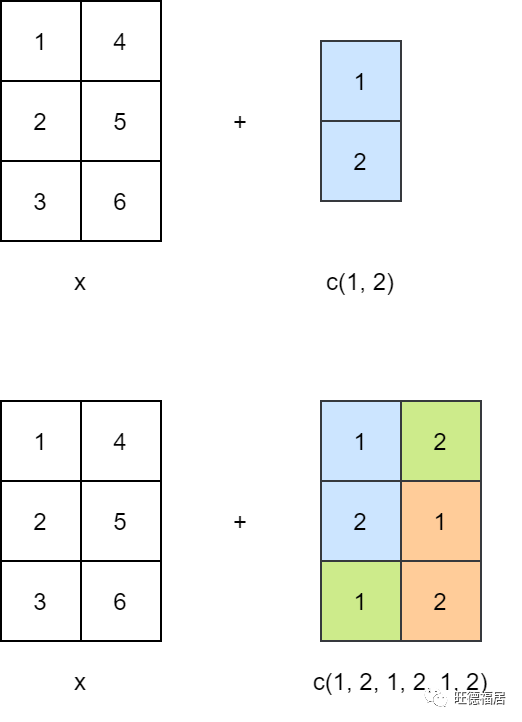print(x   [,1] [,2][1,] 2 6[2,] 4 6[3,] 4 8 常用的向量运算 向量运算和逻辑运算 R 是函数式语言，运算符也是一个函数 print(  5 print(  5 向量元素加法  c(1, 2, 4)print(x + c(5, 0, -1))  6 2 3 向量元素乘法 print(x   5 0 -4 其他元素运算  c(1, 2, 4)print(x / c(5, 4, -1))  0.2 0.5 -4.0 print(x   1 2 0 向量索引 向量1[向量2]  c(1.2, 3.9, 0.4, 0.12)print(y[c(1, 3)])  1.2 0.4 print(y[2  3.9 0.4  3:4print(y[v])  0.40 0.12 元素可以重复  c(4, 2, 17, 5)print(y x[c(1, 1, 3)])  4 4 17 负数下标代表把相应的元素剔除 注：Python 中负数下标表示反向索引  c(5, 12, 13)print(z[-1])  12 13 print(z[  13 选择除最后一个元素外的其余元素  c(5, 12, 13)print(z[1:(length(z)-1)])  5 12 print(z[  5 12 用 : 运算符创建向量 print(  5 6 7 8 print(  5 4 3 2 1 运算符优先级 注意：?Syntax 可以查看运算符优先级  2print(1:i-1)  0 1 print(  1 使用 seq() 创建向量 print(  12 15 18 21 24 27 30 注：与 Python 中不同，seq 包含区间结尾 print(   1.1 1.2 1.3 1.4 1.5 1.6 1.7 1.8 1.9 2.0 seq 可以处理空向量  c(5, 12, 13)print(x)  5 12 13 print(  1 2 3  NULLprint(x) NULL print( integer(0) 使用 rep() 重复向量常数 rep (repeat) 将同一向量重复放到长向量中  rep(8, 4)print(x)  8 8 8 8 print(  5 12 13 5 12 13 5 12 13 print(  1 2 3 1 2 3 rep() 函数的 each 参数指定交替重复的次数 print(  5 5 12 12 13 13 使用 all() 和 any() any() 参数是否至少一个为 TRUE all() 参数是否全部为 TRUE  1:10print(any(x > 8))  TRUE print(  FALSE print(  TRUE any 或 all 函数执行两步操作。 计算表达式，得到 bool 向量判断向量中是否有 TRUE 或全部为 TRUE  (x > 8)print(flag)   FALSE FALSE FALSE FALSE FALSE FALSE FALSE FALSE TRUE TRUE print(  TRUE 扩展案例：寻找连续出现 1 的游程 在 0，1 组成的序列中，一个由连续的 0 或 1 构成的串称为一个游程 (run)  function(x, k) { n length(x) runs NULLfor (i in 1:(n-k+1)) {if (all(x[i:(i+k-1)]==1)) { runs c(runs, i) } }return(runs)}y c(1, 0, 0, 1, 1, 1, 0, 1, 1)print(findruns(y, 3))  4 print(  4 5 8 print( NULL 上述代码中，每次更新 runs 向量执行的 runs 都会创建新的向量，分配内存空间。对于大型数据集，执行太多次可能会拖慢运行速度。 一种解决方法是预先分配内存空间，在返回时“删掉”不需要的元素  function(x, k) { n length(x) runs vector(length=n) count 0for (i in 1:(n-k+1)) {if (all(x[i:i+k-1] == 1)) { count count + 1 runs[count] i } }if (count > 0) { runs runs[1:count] } else { runs NULL }return(runs)} 使用 1,000,000 个数据进行测试，可以发现 findruns1 函数速度提升很明显  round(runif(1000000, min=0, max=1)) system.time(  user system elapsed 15.01 4.16 19.17 system.time(  user system elapsed 0.47 0.00 0.47 扩展案例：预测离散值时间序列 1 代表有雨，0 代表无雨。假设有一个 k 值，根据最近 k 天的记录预测明天是否会下雨。采用“过半数规则” (majority rule)，如果最近 k 个数据中 1 的个数大于等于 k/2，则预测下一个值为 1，否则为 0。 构造一个 500 个数据的随机数组  round(runif(500, min=0, max=1))print(rains[1:10])   0 0 1 0 0 0 1 0 0 1 编写第一个函数  function(x, k) { n length(x) k2 k/2 pred vector(length=n-k)for (i in 1:(n-k)) {if (sum(x[i:(i+k-1)]) >= k/2 ) { pred[i] 1 } else { pred[i] 0 } }return(mean(abs(pred-x[(k+1):n])))} 该函数返回的是 MAE (Mean Absolute Error) 使用 k=1, 2, 4 测试 print(  0.5410822 print(  0.4959839 print(  0.5 上面的函数每个循环都会计算整个子序列的和。相邻两个子序列仅开头和结尾数据不同。 编写函数，重复利用上一步的计算结果。每次循环只执行一次加法和一次减法  function(x, k) { n length(x) k2 k/2 pred vector(length=n-k) sm sum(x[1:k])if (sm > k2) pred 1 else pred 0if (n - k >= 2) {for (i in 2:(n - k)) { sm sm + x[i+k-1] - x[i-1]if (sm > k2) pred[i] 1 else pred[i] 0 } }return(mean(abs(pred-x[(k+1):n])))}print(preda(rains, 4))  0.5 另一种方法是使用累加和 cumsum() 函数代替 sum 求和  c(5, 2, -3, 8)print(cumsum(y))  5 7 4 12 编写函数，每次循环中仅使用一次减法  function(x, k) { n length(x) k2 k/2 pred vector(length=n-k) csx c(0, cumsum(x))for (i in 1:(n-k)) {if (csx[i+k] - csx[i] >= k2) { pred[i] 1 } else { pred[i] 0 } }return(mean(abs(pred-x[(k+1):n])))}print(preda(rains, 4))  0.5 测试下几种方法的耗时  round(runif(1000000, min=0, max=1)) system.time(  user system elapsed 0.79 0.00 0.79 system.time(  user system elapsed 0.17 0.01 0.18 system.time(  user system elapsed 0.14 0.00 0.14 向量化运算符 提高 R 代码执行效率的有效方法之一就是向量化 (vectorize)，即应用到向量上的函数实际上是应用到向量的每一个元素上。 向量输入、向量输出  c(5, 2, 8)v c(1, 3, 9)print(u > v)  TRUE FALSE FALSE 如果一个函数使用向量化的运算符，那么它也被向量化了，从而使速度提升成为可能。  function(x) return(x+1)print(w(u))  6 3 9 超越函数也是向量化的 print(  1.000000 1.414214 1.732051 2.000000 2.236068 2.449490 2.645751 2.828427 3.000000 许多内置 R 函数都是向量化的  c(1.2, 3.9, 0.4)z round(y)print(z)  1 4 0 print(  1 + 也是函数  c(12, 5, 13)print(y + 4)  16 9 17 print(  16 9 17 R 对向量的循环补齐可能会给期望使用标量的函数带来问题  function(x, c) {return ((x+c)^2)}print(f(1:3, 0))  1 4 9 print(  4 9 16 参数 c 也可以接收多元向量 print(  4 16 36 想将 c 限制为标量，需要加入判断语句  function(x, c) {if (length(c) != 1) stop("vector c not allowed")return ((x+c)^2)} 再次执行上面的语句 print( 会抛出错误 Error in f(1:3, 1:3): vector c not allowedTraceback:1. print(f(1:3, 1:3))2. f(1:3, 1:3)3. stop("vector c not allowed") # at line 2 of file  向量输入，矩阵输出  function(z) return(c(z, z^2))print(z12(5))  5 25  1:8print(z12(x))   1 2 3 4 5 6 7 8 1 4 9 16 25 36 49 64 以矩阵的形式更好理解，使用 matrix 函数将向量转为矩阵 print(  [,1] [,2][1,] 1 1[2,] 2 4[3,] 3 9[4,] 4 16[5,] 5 25[6,] 6 36[7,] 7 49[8,] 8 64 可以使用 sapply() (simple apply) 简化这一步骤。 调用 sapply(x, f) 会对 x 中的每一个元素应用 f()，并将结果转成矩阵。每个元素的结果作为一列 print(  [,1] [,2] [,3] [,4] [,5] [,6] [,7] [,8][1,] 1 2 3 4 5 6 7 8[2,] 1 4 9 16 25 36 49 64 NA 与 NULL 值 NA 表示缺失值 NULL 表示不存在的值 NA 的使用 统计函数支持跳过 NA 值 注：需要手动指定参数  c(88, NA, 12, 168, 13)print(x)  88 NA 12 168 13 print(  NA print(  70.25 R 会自动跳过 NULL 值  c(88, NULL, 12, 168, 13)print(x)  88 12 168 13 print(  70.25 NA 的模式与向量中其它元素保持一致  c(5, NA, 12)print(mode(x))  "numeric" print(  "numeric"  c("abc", "def", NA)print(mode(y))  "character" print(  "character" NULL 的使用 NULL 是 R 的一种特殊对象，没有模式 注：NULL 可以当成一种空指针  NULLfor (i in 1:10) {if (i %% 2 == 0) { z c(z, i) }}print(z)  2 4 6 8 10 使用 seq 也可以实现上面的功能 print(  2 4 6 8 10 或者另一种方法 print(  2 4 6 8 10 如果将上例中的 NULL 换成 NA，则不会得到想要的结果  NAfor (i in 1:10) {if (i %% 2 == 0) { z c(z, i) }}print(z)  NA 2 4 6 8 10 下面的示例可以看到，NULL 被当成不存在  NULLprint(length(u))  0  NAprint(length(v))  1 筛选 筛选 (filtering) 使我们可以提取向量中满足一定条件的元素 生成筛选索引 一个简单的示例  c(5, 2, -3, 8)w z[z*z > 8]print(w)  5 -3 8 逐步研究筛选如何实现  c(5, 2, -3, 8)print(z)  5 2 -3 8 print(z  TRUE FALSE TRUE TRUE 注意：* 和 > 都是向量运算符，z 和 8 都是向量 所以上式又可以写成 print(  TRUE FALSE TRUE TRUE 返回的结果是布尔值向量，用布尔值向量实现筛选 print(z  5 -3 8 注：numpy 中也有类似的筛选操作 筛选可以用于其它向量  c(5, 2, -3, 8)j z*z > 8print(j)  TRUE FALSE TRUE TRUE  c(1, 2, 30, 5)print(y[j])  1 30 5 或者写成更简洁的形式  c(5, 2, -3, 8)y c(1, 2, 30, 5)print(y[z*z > 8])  1 30 5 使用 subset 函数筛选 subset 与普通筛选类似，但会忽略 NA 值  c(6, 1:3, NA, 12)print(x)  6 1 2 3 NA 12 print(x[x   6 NA 12 print(  6 12 选择函数 which() which() 返回满足条件元素所在的位置  c(5, 2, -3, 8)print(which(z*z > 8))  1 3 4 了解 which 如何工作 print(z   TRUE FALSE TRUE TRUE which() 函数报告向量中哪些元素为 TRUE。 下面考虑一个简单的函数：找到满足一定条件的元素首次出现的位置。 上一篇文章中使用 for 循环查找  function(x) {for (i in 1:length(x)) {if (x[i] == 1) { break } }return(i)} 使用 which 的版本 注意：这里找出所有的 1，实际上仅需要找到第一个 1  function(x) return(which(x == 1)) 向量化的 ifelse() 函数 if 语句的向量化版本：ifelse(b, u, v) 如果 b[i] 为真，第 i 个元素为 u[i]，否则为 v[i]。  1:10y ifelse(x %% 2 == 0, 5 ,12)print(y)  12 5 12 5 12 5 12 5 12 5  c(5, 2, 9, 12)print(ifelse(x > 6, 2 * x, 3 * x))  15 6 18 24 扩展案例：度量相关性 向量 x 和 y 是时间序列变量，比如是温度和气压的观测值。定义两者的相关性为 x 和 y 同时上升或下降次数占观测数的比例。即 y[i+1] - y[i] 与 x[i+1] - x[i] 符号相同时的次数占总数 i 的比例。  function(v) { vud v[-1] - v[-length(v)]return (ifelse(vud >0, 1, -1))} 示例 print(  1 1 -1 1 -1 1 1 1 -1 1 udcorr 计算相关性  function(x, y) { ud lapply(list(x, y), findud)return (mean(ud[]==ud[]))} 示例  c(5, 12, 13, 3, 6, 0, 1, 15, 16, 8, 88)y c(4, 2, 3, 23, 6, 10, 11, 12, 6, 3, 2)print(udcorr(x, y))  0.4 其中 lapply() 返回一个列表 注意：R 语言中的列表类似 Python 中的字典 print( []  1 1 -1 1 -1 1 1 1 -1 1[]  -1 1 1 -1 1 1 1 -1 -1 -1 更高级的版本使用 diff 函数，对向量做“滞后”运算，默认滞后一个元素，即与前一个元素做差  c(1, 6, 7, 2, 3, 5)print(diff(u))  5 1 -5 1 2 sign 函数根据数值正负返回 1, 0 或 -1  c(1, 6, 7, 2, 3, 5)print(diff(u))print(sign(diff(u)))  5 1 -5 1 2 1 1 -1 1 1 使用上面两个函数，可以将 udcorr 函数写为一行  function(x, y) mean(sign(diff(x)) == sign(diff(y))) 测试  c(5, 12, 13, 3, 6, 0, 1, 15, 16, 8, 88)y c(4, 2, 3, 23, 6, 10, 11, 12, 6, 3, 2)print(udcorr(x, y))  0.4 扩展案例：对鲍鱼数据集重新编码 ifelse 可以嵌套使用。 在鲍鱼数据集中，性别被编码为 M，F 或 I (Infant，表示幼虫)。 假设少量样本的性别被保存在 g 中，将字符变量重新编码为 0, 1, 2  c("M", "F", "F", "I", "M", "M", "F")print(ifelse(g == "M", 1, ifelse(g == "F", 2, 3)))  1 2 2 3 1 1 2 使用 args 参数查看形式参数名字 args(ifelse) function (test, yes, no)NULL R 语言使用“惰性求值” (lazy evaluation)，只有当需要时表达式才会被计算，否则不计算。 这意味着上述两重 ifelse 不是对全部数据都进行两次计算，而是只有对第一次计算为 FALSE 的值，才会进行第二次计算。 可以使用 which 寻找不同性别数据的编号  which(g == "M")print(m)  1 5 6  which(g == "F")print(f)  2 3 7  which(g == "I")print(i)  4 可以将这些子集保存到列表中 注意：for 循环支持字符串向量  list()for (gen in c("M", "F", "I")) { grps[[gen]] which(g == gen)}print(grps) $M 1 5 6$F 2 3 7$I 4
为鲍鱼数据集添加表头
 c("Gender", "Length", "Diameter", "Height", "WholeWt", "ShuckedWt", "ViscWt", "ShellWt", "Rings")
使用 read.csv 载入数据集
 read.csv("Abalone.data",    header=FALSE,    col.names=title,    as.is=TRUE)print(head(aba))
  Gender Length Diameter Height WholeWt ShuckedWt ViscWt ShellWt Rings1      M  0.455    0.365  0.095  0.5140    0.2245 0.1010   0.150    152      M  0.350    0.265  0.090  0.2255    0.0995 0.0485   0.070     73      F  0.530    0.420  0.135  0.6770    0.2565 0.1415   0.210     94      M  0.440    0.365  0.125  0.5160    0.2155 0.1140   0.155    105      I  0.330    0.255  0.080  0.2050    0.0895 0.0395   0.055     76      I  0.425    0.300  0.095  0.3515    0.1410 0.0775   0.120     8
分雌雄两组，对直径和长度作图
 list()for (gen in c("M", "F", "I")) {    grps[[gen]]  which(aba[,1] == gen)}abam  aba[grps$M,]abaf aba[grps$F,]plot(abam$Length, abam$Diameter)plot(abaf$Length, abaf$Diameter, pch="x", new=FALSE)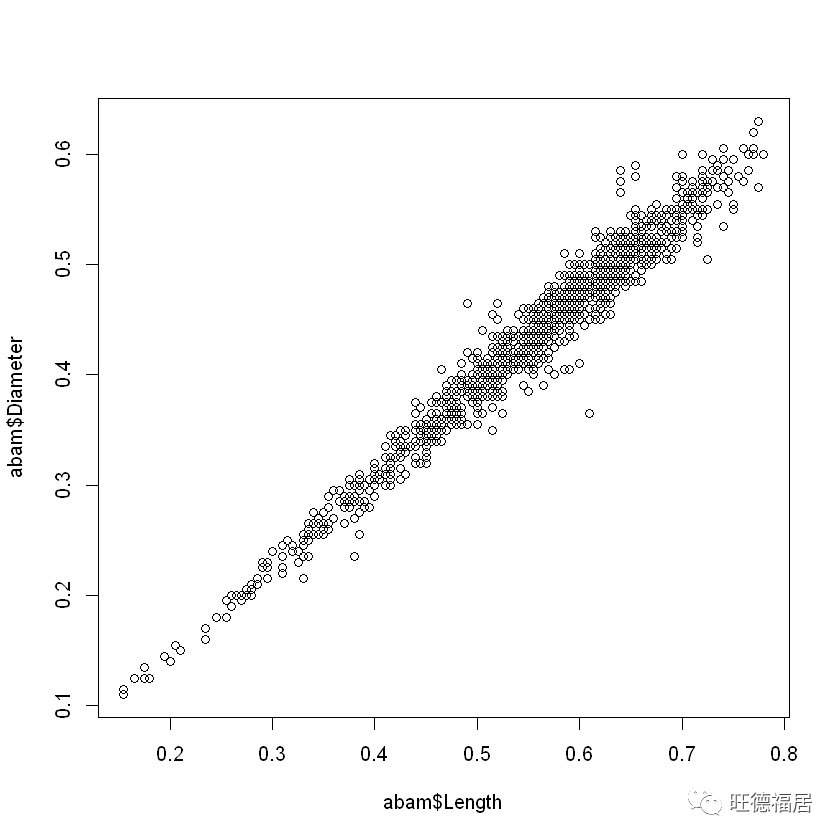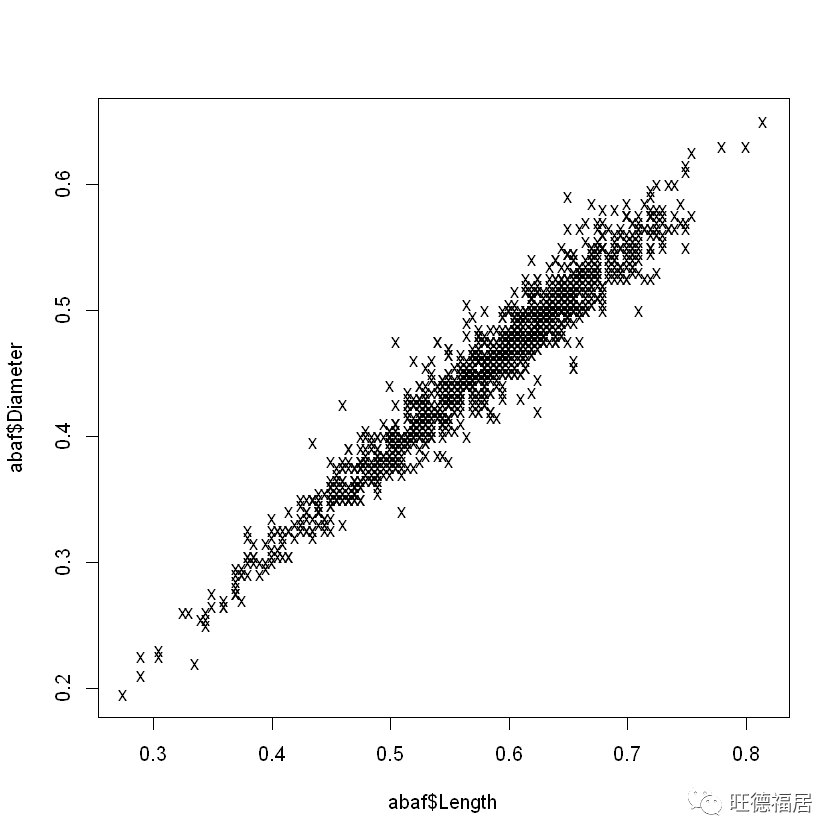pch 参数可以是向量
 ifelse(aba$Gender == "M", "o", "x")plot(aba$Length, aba$Diameter, pch=pchvec)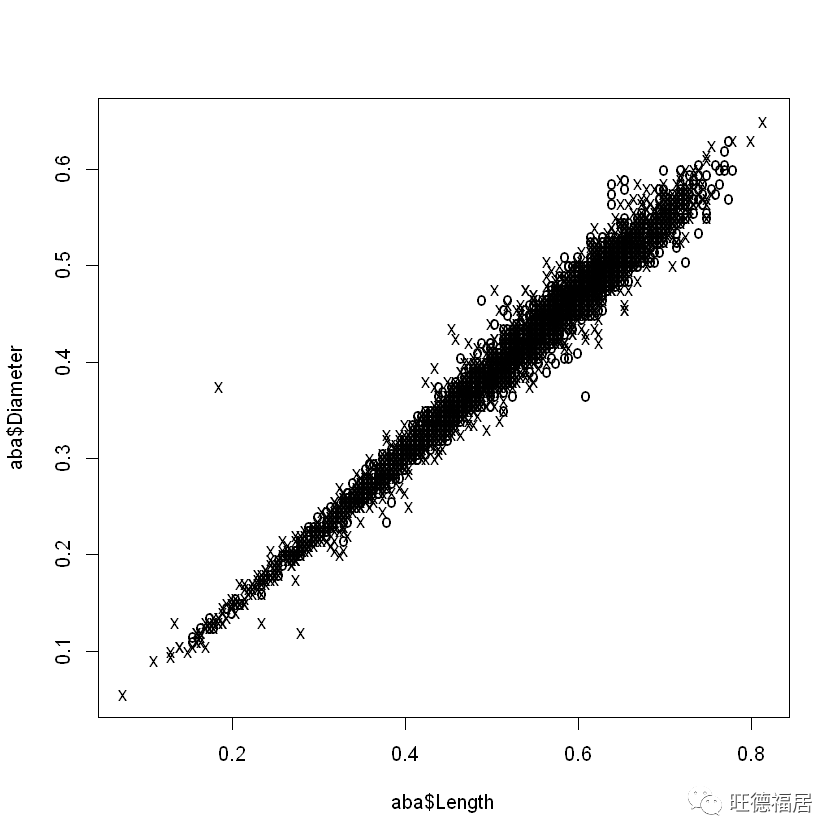测试向量相等 因为 == 是向量化函数，使用 == 比较两个向量是否相等不会得到预想的结果  1:3y c(1, 3, 4)print(x == y)  TRUE FALSE FALSE 一种可行的方法是结合 all 函数使用 == 运算符 print(  FALSE 另一种方法是使用 identical 函数 print(  FALSE identical 函数判断两个对象是否完全一致。 下面是一个值得 思考 的示例  1:2print(x)  1 2  c(1, 2)print(y)  1 2 print(  FALSE print(  "integer" print(  "double" : 产生的是整数，c() 产生的是浮点数 向量元素的名称 可以给向量元素随意指定名称  c(1, 2, 4)names(x) NULL names(x)   "a" "b" "ab" "a"   a b ab 1 2 4 将向量名称赋值为 NULL，可以将其删除 names(x)   1 2 4 可以使用名称来引用向量中的元素  c(1, 2, 4)names(x) c("a", "b", "c")print(x["b"]) b2 注：向量名称可以使用任意值，比如列表  c(1, 2, 4)names(x) list(c(1, 2), "c", TRUE)print(x) c(1, 2) c TRUE 1 2 4 关于 c() 的更多内容 c() 会自动将不同类型的参数降级为同一类型 print(  "5" "2" "abc" print( [] 5[] 2$a 1$b 4 c() 对向量有扁平化效果 print(  5.0 2.0 1.5 6.0 注：Python 中类似的操作会生成嵌套列表 参考 《学习 R 语言：快速入门》题图由 Emmi Nummela 在 Pixabay 上发布。  展开全文• ' ', 'w', 'o', 'r', 'l', 'd'] np.c_[X] array([['h'], ['e'], ['l'], ['l'], ['o'], [' '], ['w'], ['o'], ['r'], ['l'], ['d']], dtype=' You can also do (note the extra square brackets) >>> np.array([X])....I was wondering if there's a function that takes a list X as input and outputs a column vector corresponding to X? (I looked around and I suspect it might be: np.matrix(X).T ) 解决方案 I don't think there is a function, but there is a dedicated object, np.c_ >>> X = list('hello world') >>> X ['h', 'e', 'l', 'l', 'o', ' ', 'w', 'o', 'r', 'l', 'd'] np.c_[X] array([['h'], ['e'], ['l'], ['l'], ['o'], [' '], ['w'], ['o'], ['r'], ['l'], ['d']], dtype=' You can also do (note the extra square brackets) >>> np.array([X]).T  展开全文• 1.R简介 R是S语言的一种实现。S语言是由 AT&T贝尔实验室开发的一种用来 进行数据探索、统计分析、作图的...后来Auckland大学的 RobertGentleman Ross Ihaka 及其他志愿人员开发了一个R系 统。R的使用与S-PLU  1.R简介 R是S语言的一种实现。S语言是由 AT&T贝尔实验室开发的一种用来 进行数据探索、统计分析、作图的解释型语言。最初S语言的实现版 本主要是S-PLUS。S-PLUS是一个商业 软件，它基于S语言，并由 MathSoft公司的统计科学部进一步完善。后来Auckland大学的 RobertGentleman 和 Ross Ihaka 及其他志愿人员开发了一个R系 统。R的使用与S-PLUS有很多类似之处，两个软件有一定的兼容性。 R是用于统计分析、绘图的语言和操作环境。R是属于GNU系统的一个自由、免费 、源代码开放的软件，它是一个用于统计计算和统计制图的优秀工具。 R是一套完整的数据处理、计算和制图软件系统。其功能包括：数据存储和处理系 统；数组运算工具（其向量、矩阵运算方面功能尤其强大）；完整连贯的统计分析工 具；优秀的统计制图功能；简便而强大的编程语言：可操纵数据的输入和输入，可实 现分支、循环，用户可自定义功能。 R是一个免费的自由软件，它有UNIX、LINUX、MacOS和WINDOWS版本，都是 可以免费下载和使用的,在那儿可以下载到R的安装程序、各种外挂程序和文档。在R的 安装程序中只包含了8个基础模块，其他外在模块可以通过CRAN获得。 R的特点 1．有效的数据处理和保存机制。 2．拥有一整套数组和矩阵的操作运算符。 3．一系列连贯而又完整的数据分析中间工具。 4．图形统计可以对数据直接进行分析和显示，可用于多种图形设备。 5．一种相当完善、简洁和高效的程序设计语言。它包括条件语句、循环语句、用户自 定义的递归函数以及输入输出接口。 6．R语言是彻底面向对象的统计编程语言。 7．R语言和其它编程语言、数据库之间有很好的接口。 8．R语言是自由软件，可以放心大胆地使用，但其功能却不比任何其它同类软件差。 9．R语言具有丰富的网上资源 2.向量 2.1 向量定义 具有大小和方向的量 2.2 生成向量： 2.2.1 c() > c(1,4,3,5,6)  1 4 3 56 2.2.2 a:b > 1:7  1 2 3 4 5 6 7 这两个效果都是产生向量 2.2.3 seq() >seq(2,10) 产生向量元素从2到10的向量  2 3 4 5 6 7 8 9 10 >seq(2,10,by=2) 产生从2到10间隔为2的向量元素  2 4 6 8 10 间隔是2 生成的向量的元素是2+2n<=10 其中n=(0,1,2……) 如果没有by=2,那么缺省值by=1 >seq(2,10,length=6) 从2到10均匀产生6个向量元素  2.0 3.6 5.2 6.8 8.4 10.0 一共产生6个向量元素 其中第一个为向量元素为2，最后一个向量元素10，从2到10均分成6份 这个函数的by属性和length属性不能共存 这俩属性有冲突 如 ：上面两个例子 2.2.4 letters 它不是一个函数 是26字母的数据集 多余的用NA表示 >letters[1:26] 产生26英文字母  "a" "b""c" "d" "e" "f" "g""h" "i" "j" "k" "l""m" "n" "o" "p" "q" "r"  "s" "t""u" "v" "w" "x" "y""z" >letters[1:30]  "a" "b""c" "d" "e" "f" "g""h" "i" "j" "k" "l""m" "n" "o" "p" "q" "r""s" "t" "u" "v" "w""x" "y"  "z" NA NA NA NA 产生26个英文字母，多余的用NA表示 2.3 向量操作 2.3.1 which() > x=seq(2,20,3) 产生从2到20间隔为3的向量元素 > x  2 5 811 14 17 20 >which.max(x) which.max(x)返回最大元素坐标  7 >which.min(x) which.min(x)返回最小元素下标  1 >which(x==11) which(x==11)返回向量元素为11的元素下标  4 >which(x>11) which(x>11)返回向量元素大于11的元素下标  5 6 7 > which(x)函数返回向量元素下标 2.3.2 rev() 向量的元素逆置 > x=c(1,4,3,2,6,8) > x  1 4 3 2 6 8 > rev(x)  8 6 2 3 4 1 > 2.3.3 sort() 向量的元素从小到大排序 > x=c(1,4,3,2,6,8) > x  1 4 3 2 6 8 > sort(x)  1 2 3 4 6 8 补： 数组 一系列标量的集合，在R中是指数字的集合在R中先生成向量然后在转换成数组 X=c(1:10)生成一个向量 用dim(x)<-c(2,5) 其中c中的2是生成数组的行5是生成数组的列 > x=c(1:10) > is.array(x) FALSE x不是数组 > dim(x)=c(2,5) 将x转化成一个二维数组 > is.array(x) x是数组  TRUE > x [,1] [,2] [,3] [,4] [,5] [1,] 1 3 5 7 9 [2,] 2 4 6 8 10 > 生成一个二维数组  展开全文• R中，基本的数据结构有：向量，矩阵，数组，数据框，列表，因子，函数等。 向量：一系列同类型的有序元素构成。 向量是一维结构。 向量R最简单的数据结构，在R中没有标量。... 矩阵需要输入行数，数... • 本文，是对R语言向量的总结，适合新手入门，老手巩固总结，顺便强调一下R语言向量的重要性，向量被誉为R语言中的战斗机。希望你阅读完本文后，能对向量有一个新的理解！文章内容有点多，这是因为我把一些晦涩... • IRIS数据集简介 IRIS数据集中的数据源于1936年费希尔法发表的一篇论文。彼时他收集了三种鸢尾花（分别标记为setosa、versicolor...有关数据可以从datasets软件包中的iris数据集里获取，下面我们演示性地出了...机器学习 • 有时候我们在使用matlab的时候，想对向量或者矩阵进行转置，怎么转置呢，下面来分享一下方法工具/材料matlab向量和矩阵转置方法向量转置方法01第一步在我们的电脑上打开matlab，在命令行窗口中输入r = [ 1 2 3 4 ]... • 02 R语言入门——向量、矩阵与函数 2.1 对象(objects) 2.2 元素的类型 2.3 运算符 2.4 对象的类 2.5 类的判断 2.6 序列和向量 2.7 向量运算 2.8 向量名字 2.9 向量的大小次序 2.10 R的函数 2.11 构建矩阵 2.12 矩阵... • 首先输入值，然后是矩阵，输出将采用向量 [rc] 的形式，其中 r 是值的，c 是。 如果该值出现多次，则输出将有更多 [rc]。 您还可以以 getcoord(COORDVAL, MATRIX, RANGEROW, RANGECOL) 形式输入函数其中 ...matlab • R的数据类型与相应运算7 R向量下标子集7.1 正整数下标7.2 负整数下标7.3 ...（学习资料参考北京大学李东风老师《R语言教程》） 7 R向量下标子集 在R中下标与子集是极为强大的功能， 需要一些练习才能熟练掌握， ... • Support Vector Machines ------------------- Step 1: Exploring and preparing the ...将输入读入到R中，确认接收到的数据具有16个特征，这些特征定义了每一个字母的案例。 letters <- read.csv("F:\\rwork... • 向量和矩阵 1.手动输入数组 (1)所有MATLAB变量都是数组: 这意味着每个变量均可以包含多个元素 单个称为标量的数值实际上是一个1×1数组 (2)创建包含多个元素的数组: #用空格/逗号(,)分隔的值会被组合为一个行向量 ...matlab 列表 • B = A(v1,v2) v1表示子矩阵要保留的行号构成的向量 v2表示要保留的列号构成的向量 : 表示要提取所有或列，取决于其位置 end的使用 B = A(1:2:end,:)//取奇数列向量 C = A([1 1 1 1 ],:)//取第一列向量，取... • R是向量化的语言，最突出的特点是对向量的运算不需要显式编写循环语句，它会自动地应用于向量的每一个元素。对象是R中存储数据的数据结构，存储在内存中，通过名称或符号访问。...R语言非常灵活，例如： ... • apply函数族包括apply(),lapply(),sapply(),vapply(),mapply(),rapply(),tapply()，在实际应用中需要依据不同数据结构数据处理目的采用不同的函数,apply函数族的向量化运算是基于C语言实现的 1.apply函数 apply...apply split • matlab 获取矩阵向量长度 length size觉得有用的话, 欢迎一起讨论相互学习~概论size: ...返回一个行向量, 该行向量的第一个元素时数组的行数, 第二个元素是数组的数.[r,c]=size(A), 当有两个输出参数时, s... • R语言作为大数据中常用到的基础软件，学习好R语言具有非常重要的作用在本部分的学习之中，我将对R语言向量、矩阵、数组、列表、数据框等R语言常用到的数据形式做出简明扼要的总结；1.向量1.1 向量的创建...数据分析 • R中的内置数据集存储在datasets这个包中，这些数据一般搜集于真实的调查数据。 help(package=“datasets”)：查看数据集包。 data()：出所有数据集。 直接输入数据集名字，就可以使用该数据集。这些内置数据集的... • （1）行向量 创建行向量括在方括号中的元素的集合，用空格或逗号分隔的元素。 >> r = [7 8 9 10 11] >>= [7,8, 9, 10, 11] r = 7 8 9 10 11 >> r = [7 8 9 10 11]; t = [2, 3, 4, 5, 6]; res = r + t ... • 向量a,b装换成一个字符串，其中： a=c(1,2,3,4) b=c(2,3,4,5) 为了将其转化成一个字符串可以通过引入包stringr，使用str_c实现，使用paste也一样可以达到目的，如下： library(stringr) a_b=str_c(a,b,... • 行向量 列向量 MATLAB 行向量： 创建行向量括在方括号中的元素的集合，用空格或逗号分隔的元素。 r = [7 8 9 10 11] 执行上述语句，返回下述结果： r = 7 8 9 10 MATLAB 列向量： 创建列向量括...matlab • 3.3 对矩阵的行和列调用函数 *apply()函数系列是R中最受欢迎同时也是最常用的，该函数系列包括apply()、tapply()lapply()。这里我们主要介绍apply()。apply()函数允许用户在矩阵的各或各上调用指定的函数。... • R语言基础：数组和列表数组(array) 一维数据是向量，二维数据是矩阵，数组是向量和矩阵的直接推广，是由三维或三维以上的数据构成的. 数组函数是array(),语法是：array(dadta, dim)，其中data必须是同一类型的数据...数组 列表 • R中常用的数据结构包括：同质数据类型（homogeneous data types），即所存储的一定是相同类型的元素，包括向量、矩阵、数组；异质数据类型（heterogeneous data types），即可以存储不同类型的元素，这大大提高了... • Matlab、R向量与矩阵操作 描 述 Matlab R 1 建立行向量v=[1 2 3 4] v=[1 2 3 4] ...-scan()，然后输入1 2 3 4，并按Enter ...建立列向量v=[1 2 3 ... • {12}&\cdots&a_{1n}\\ a_{21}&a_{22}&\cdots&a_{2n}\\ \vdots&\cdots&\ddots&\vdots&\\ a_{m1}&a_{m2}&\cdots&a_{mn}\\ \end{array} 矩阵字母表示，双R表示$\mathbb{R}^{9947*15774}\$ R9947∗15774\mathbb{R}^{...latex公式
• R是向量化的语言，最突出的特点是对向量的运算不需要显式编写循环语句，它会自动地应用于向量的每一个元素。对象是R中存储数据的数据结构，存储在内存中，通过名称或符号访问。...R语言非常灵活，例如： R...python java 数据结构 大数据 javascript...